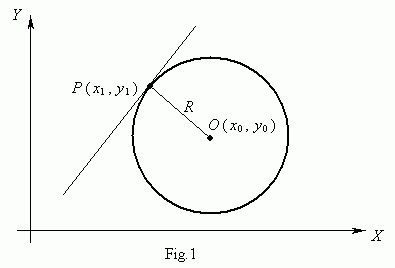Circle

Circle. Center of circle. Radius of circle.

Equation of circle. Equation of tangent line to circle.

Tangency condition of straight line and circle.

A circle ( Fig.1 ) is a locus of points, equidistant from the given point Î , called a center of circle , at the distance R . A number R > 0 is called a radius of circle .An equation of circle of radius R with a center in a point Î ( õ 0 , ó 0 ) is:

( õ õ 0 ) 2 + ( ó ó 0 ) 2 = R 2 .

If a center of the circle coincides with the origin of coordinates , then an equation of circle becomes :

õ 2 + ó 2 = R 2 .

Let Ð ( õ 1 , ó 1 ) be a point of the circle ( Fig.1 ), then an equation of tangent line to circle in the given point is:

( õ 1 õ 0 ) ( õ õ 0 ) + ( ó 1 ó 0 ) ( ó ó 0 ) = R 2 .

A tangency condition of a straight line y = m x + k and a circle õ 2 + ó 2 = R 2 :

k 2 / ( 1 + m 2 ) = R 2 .